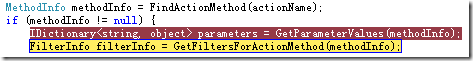# ASP.NET MVC，深入浅出IModelBinder，在Post方式下慎用HtmlHelper

```public ActionResult SimplePost(string number)
{
ViewData["Title"] = "SimplePost Page";
ViewData["Message"] = "Increase :";

#region Increase
SimplePostModel model = new SimplePostModel();
int result;

if (!string.IsNullOrEmpty(number))
{
if (int.TryParse(number, out result))
{
model.SimplePostResult = result;
ViewData["number"] = model.Increase();
}
else
{
ViewData["number"] = number;
}
}
else
{
ViewData["number"] = model.SimplePostResult;
}
#endregion

return View();
}```

```<%@ Page Language="C#" AutoEventWireup="true" MasterPageFile="~/Views/Shared/Site.Master" CodeBehind="SimplePost.aspx.cs" Inherits="MvcAppWarningPostWithHtmlHelper.Views.Home.SimplePost" %>
<%@ Import Namespace="MvcAppWarningPostWithHtmlHelper.Models" %>

<asp:Content ID="indexContent" ContentPlaceHolderID="MainContent" runat="server">
<h2>
<%= Html.Encode(ViewData["Message"]) %>h2>
<% using (Html.BeginForm("SimplePost", "Home", "post"))
{%>
<input type="text" name="number" value="<%= ViewData["number"] %>" />
<br />
<input type="submit" value="Increase" title="Update Form"/>
<%}
%>
asp:Content>````IDictionary<string, object> parameters = GetParameterValues(methodInfo);`

DefaultModelBinder通过反射的方式，利用我们提供的ActionMethod的参数名，在Form中寻找对应的名字以及它的属性。虽然我们从未提供对应属性的值，但是根据反射，即可获得对应属性名，再依照约定将这些名字组合成对应的Form Key，即可从Form中获取相应的元素值了。具体对应规则则为：

```public class User
{
public string Name { get; set; }
public int Age { get; set; }
}```

```namespace MvcAppWarningPostWithHtmlHelper.Models
{
public class UserModelBinder : IModelBinder
{
public UserModelBinder()
{
NameUniqueID = "user\$Name";
AgeUniqueID = "user\$Age";
SpouseNameUnique = "userSpouse\$Name";
SpouseAgeUnique = "userSpouse\$Age";
}
private string NameUniqueID { get; set; }
private string AgeUniqueID { get; set; }
private string SpouseNameUnique { get; set; }
private string SpouseAgeUnique { get; set; }

private User UserConvert(string name, string age, User spouse)
{
int iAge = 0;
int.TryParse(age, out iAge);
if (spouse != null && spouse.Spouse == null)
{
spouse.Spouse = new User(name, iAge, spouse);
}
return new User(name, iAge, spouse);
}

#region IModelBinder 成员

public ModelBinderResult BindModel(ModelBindingContext bindingContext)
{
HttpRequestBase request = bindingContext.HttpContext.Request;
if (request.Form != null && request.Form.HasKeys())
{
return new ModelBinderResult(UserConvert(request.Form.GetValues(NameUniqueID),
request.Form.GetValues(AgeUniqueID),
UserConvert(request.Form.GetValues(SpouseNameUnique),
request.Form.GetValues(SpouseAgeUnique),
null)));
}
return null;
}

#endregion
}
}```

```public ActionResult CollectionPost(IList<Product> models)
{
//Other code!!……
ViewData.Model = models;
return View();
}```

```<asp:Content ID="indexContent" ContentPlaceHolderID="MainContent" runat="server">
<h2>
<%= Html.Encode(ViewData["Message"]) %>h2>
<% using (Html.BeginForm("CollectionPost", "Home", "post"))
{
int current = 0;
foreach (Product item in (IEnumerable)ViewData.Model)
{
current = item.Id; %>
<input type ="hidden" name="models.index" value = "<%= current.ToString() %>"/>
<%=Html.Hidden("models[" + current.ToString() + "].Id", item.Id) %>
<%=Html.TextBox("textbox1_" + item.Id.ToString(), item.Name,
new { name = "models[" + current.ToString() + "].Name" })%>
<%=Html.TextBox("textbox2_" + item.Id.ToString(), item.Description,
new { name = "models[" + current.ToString() + "].Description" ,
style= "width:400px;"})%>
<br />
<%}%>
<br />
<input type="submit" value="Submit" title="Update Form"/>
<%}
%>
asp:Content>```

<input type ="hidden" name="models.index" value = "<%= current.ToString() %>"/>

```<form action="/?Length=4" method="post">
<input type="hidden" name="models.index" value="200" />
<input id="models.Id" name="models.Id" type="hidden" value="200" />
<input id="textbox1_200" name="models.Name" type="text" value="Product0" />
<input id="textbox2_200" name="models.Description" style="width: 400px;" type="text"
value="I am the Product0, made in China!" />
<br />
<input type="hidden" name="models.index" value="201" />
<input id="models.Id" name="models.Id" type="hidden" value="201" />
<input id="textbox1_201" name="models.Name" type="text" value="Product1" />
<input id="textbox2_201" name="models.Description" style="width: 400px;" type="text"
value="I am the Product1, made in China!" />
<br />
<input type="hidden" name="models.index" value="202" />
<input id="models.Id" name="models.Id" type="hidden" value="202" />
<input id="textbox1_202" name="models.Name" type="text" value="Product2" />
<input id="textbox2_202" name="models.Description" style="width: 400px;" type="text"
value="I am the Product2, made in China!" />
<br />
<input type="hidden" name="models.index" value="203" />
<input id="models.Id" name="models.Id" type="hidden" value="203" />
<input id="textbox1_203" name="models.Name" type="text" value="Product3" />
<input id="textbox2_203" name="models.Description" style="width: 400px;" type="text"
value="I am the Product3, made in China!" />
<br />
<input type="hidden" name="models.index" value="204" />
<input id="models.Id" name="models.Id" type="hidden" value="204" />
<input id="textbox1_204" name="models.Name" type="text" value="Product4" />
<input id="textbox2_204" name="models.Description" style="width: 400px;" type="text"
value="I am the Product4, made in China!" />
<br />
<br />
<input type="submit" value="Submit" title="Update Form" />
form>```

```<%--<%=Html.Hidden("models.Index", current.ToString()) %>--%>
<input type ="hidden" name="models.index" value = "<%= current.ToString() %>"/>```

<input id="models.Id" name="models.Id" type="hidden" value="200" />

<input id="models.Id" name="models.Id" type="hidden" value="200,201,202,203,204" />

```if (isCheckBox) {
// Helpers that take isChecked as parameter should never look at ViewData
if (useViewData) {
isChecked = htmlHelper.EvalBoolean(name);
}
tagBuilder.MergeAttribute("value", Convert.ToString(value, CultureInfo.CurrentUICulture));
}
else {
tagBuilder.MergeAttribute("value", attemptedValue ?? ((useViewData) ? htmlHelper.EvalString(name) : Convert.ToString(value, CultureInfo.CurrentUICulture)));
}```

1、回发，也就是数据有机会叠加的情况；

2、重复name，也就是从Form[name]能取出一个集合而不是单一元素的时候发生。

0
0